# Many Ways to MultiplyMultiplication can challenge young mathematicians, and memorizing basic facts is a major pain point for some. Developing a strong conceptual understanding of what it means to multiply will help kids master facts more easily.

Beginning in second grade, students are introduced to multiplication through repeated addition and arrays. This foundation is essential as students progress to multiplying multi-digit numbers beginning in third grade. Different approaches to multiplying multi-digit numbers include the grid method (sometimes called the box method or area model) and the standard algorithm (sometimes called long multiplication).

These approaches can help students develop a strong conceptual understanding of multiplication.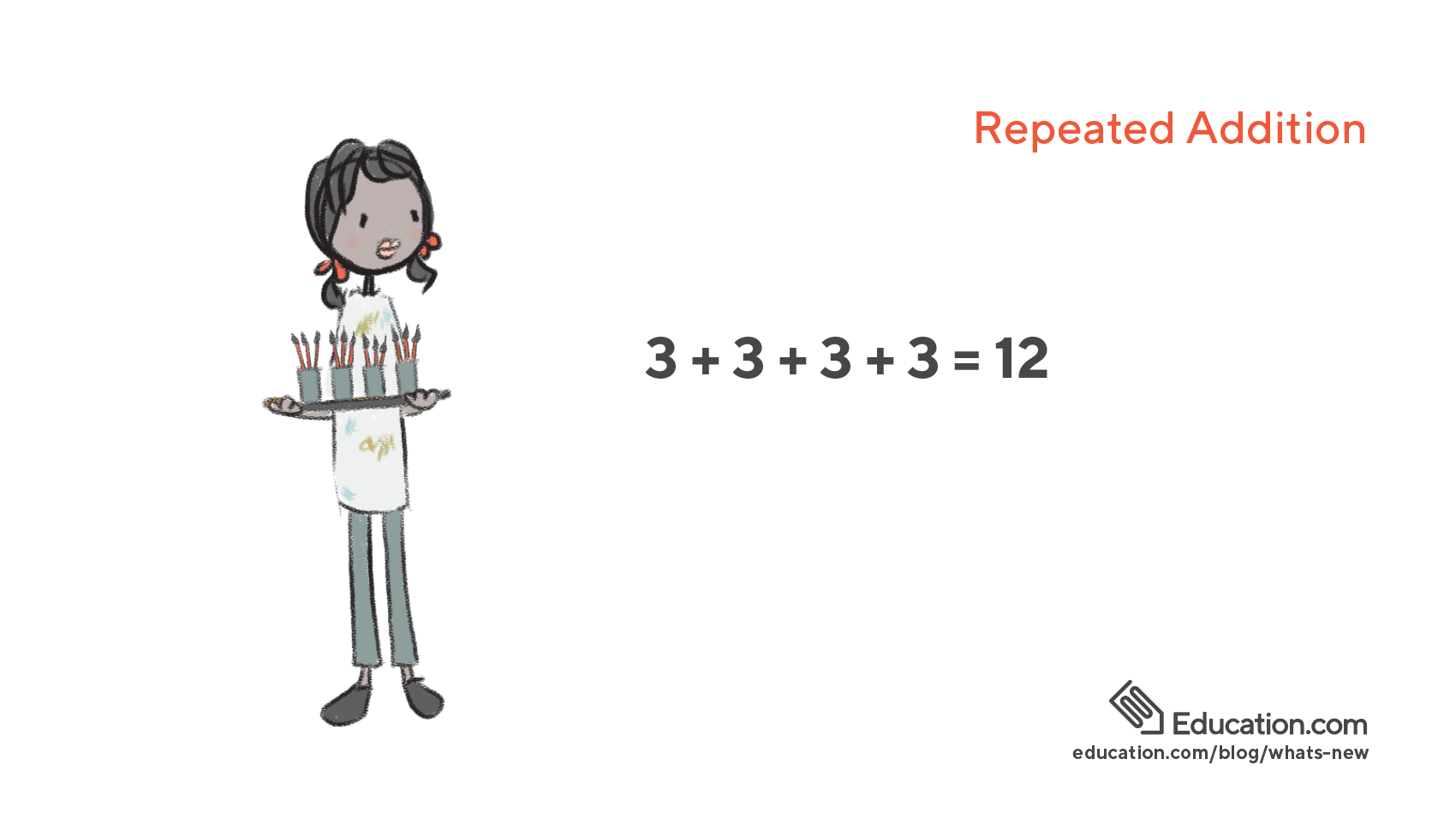Early exposure to multiplication will include problems that require repeated addition. Consider the problem: Jenny has four cups. There are three paint brushes in each cup. How many paint brushes does Jenny have in all?

This problem asks students to add equal sized sets repeatedly: 3 + 3 + 3 + 3 = 12.

Exposing kids to problems that require adding equal sized sets repeatedly will help them to conceptualize multiplication in concrete ways. Students will learn later that another way to solve the problem is 3 x 4 = 12.

For more practice with repeated addition, try out this worksheet.

Arrays

An array is a simple arrangement of objects into rows and columns that helps children visualize multiplication. An array for the example problem above would appear like this: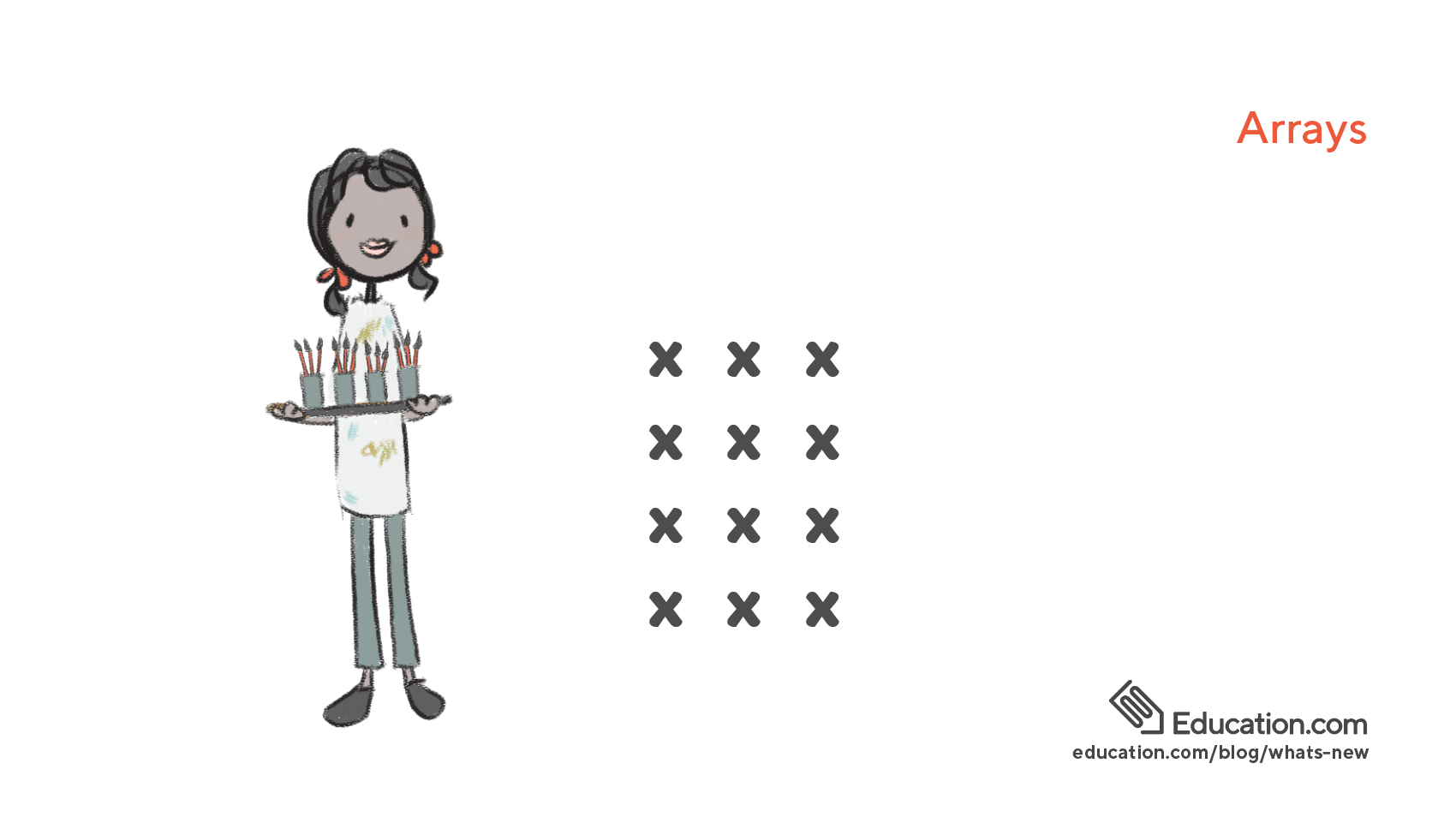This array makes it easy to see that there are 3 columns and 4 rows (3 x 4 = 12).

Arrays are a helpful way to represent multiplication as numbers get bigger.

To use an array to multiply:

1. Print a copy of the 5 x 5 array found here.
2. Practice multiplication facts to 25 by covering lines and rows of dots to show different multiplication problems. Cover the bottom row and two left columns to practice the fact 3 x 4.
3. Talk about how the array shows repeated addition. Reveal another column of dots to show that 4 groups of 4 dots (4 x 4) equals 16, which is the same as 12 + 4 = 16.
4. Reorient the array by flipping it on its side to show that 3 x 4 and 4 x 3 both equal 12. This lays a foundation for children to understand the commutative property.
5. Introduce a 10 x 10 dot array to support kids as they memorize their multiplication facts to 100!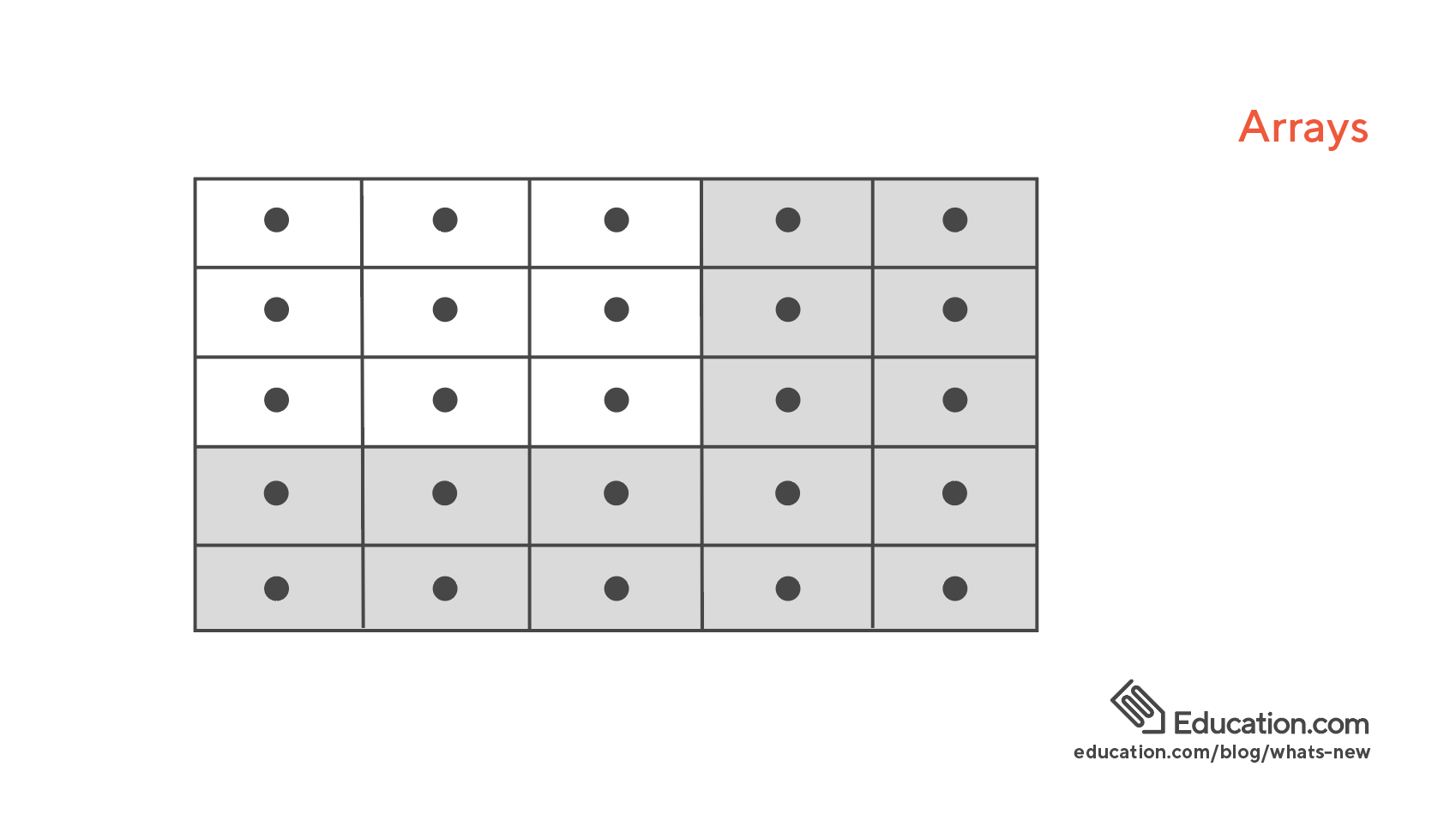For more practice multiplying with arrays play the game Candy Shop Arrays.

The Grid Method

Also sometimes called the Box Method or Area Model, the Grid Method helps kids develop number sense by decomposing each factor in the multiplication problem.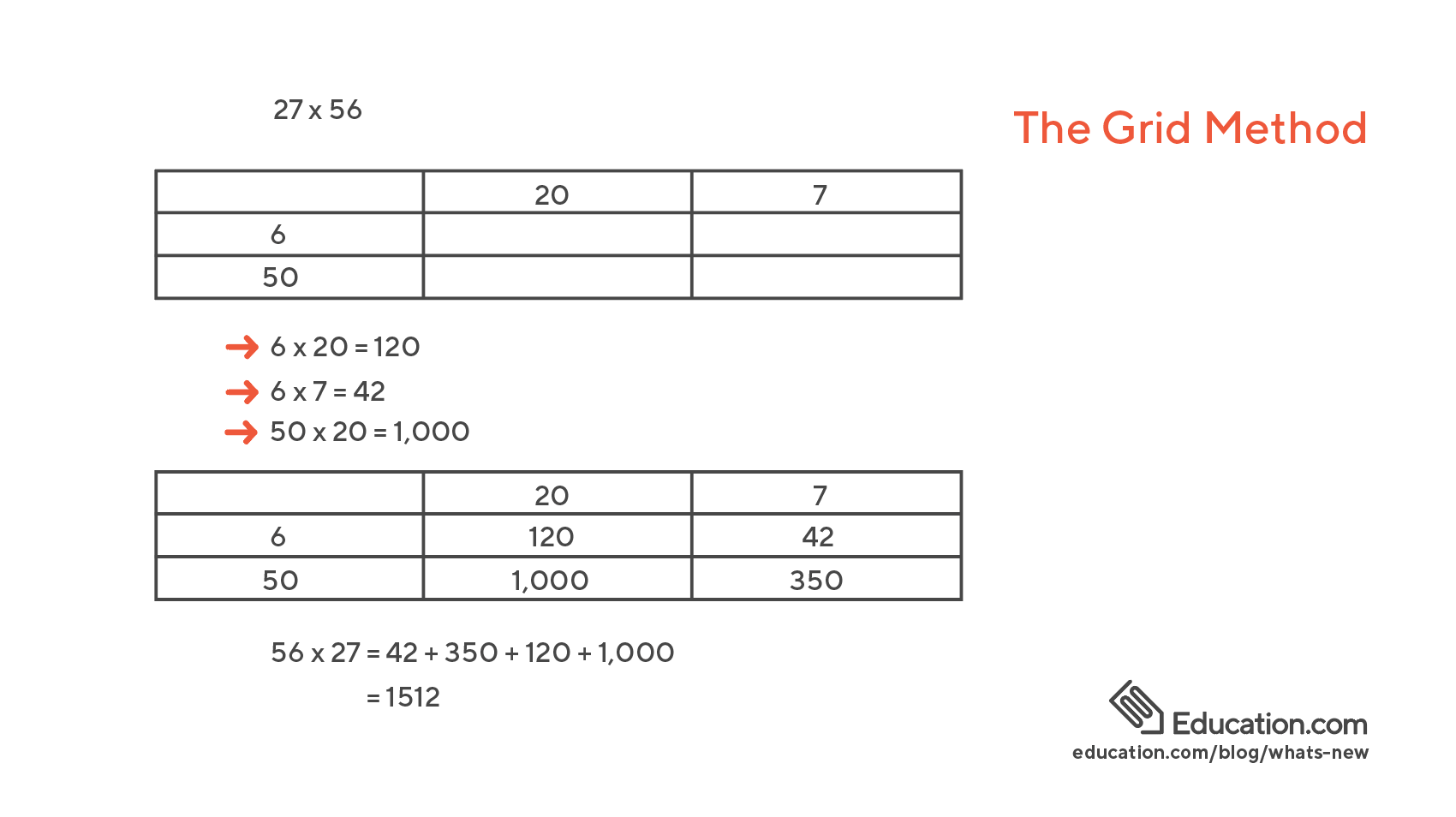Follow these steps to solve the problem 27 x 56:

1. Represent the factor 27 as 20 + 7. 56 is represented as 50 + 6.
2. Create a grid, or box:
3. Multiply each number in the left column with each number on the top row, solving for four “partial products.”

6 x 20 = 120
6 x 7 = 42
50 x 20 = 1,000
50 x 7 = 350

1. Write each number on the grid.
2. Add the 4 partial products. Here, 56 x 27 = 42 + 350 + 120 + 1,000. So, 56 x 27 = 1512.

Watch this video for more examples of multiplying using partial products.

Standard Algorithm

The standard algorithm, also called long multiplication, is handy because it makes it possible to multiply numbers of any size.

Follow these steps to multiply 56 x 27: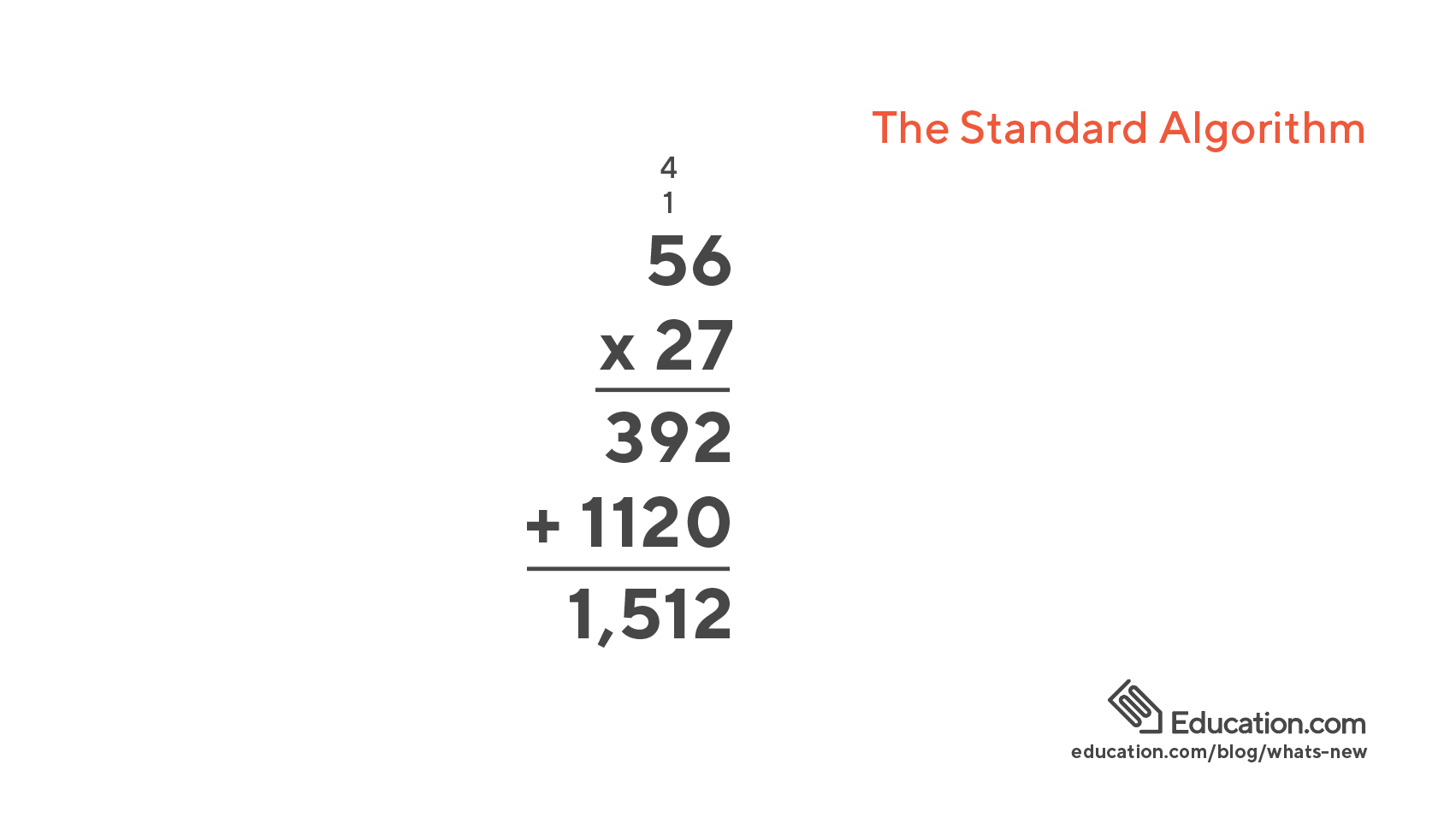1. Starting on the left, multiply 7 x 6 = 42. Write 2 below the 7, and carry the 4. Multiply 7 x 5 = 35. Add 4 to calculate the first partial product, 392.
2. Write a 0 below the 2. Multiply 2 x 6 = 12. Write 2 to the left of the 0, and carry the 1. Multiply 2 x 5 =10. Add 1 to calculate the second partial product 1,120.
3. Add the partial products. 392+ 1,1 20 = 1,512.

Watch this video for more examples of how to multiply using the standard algorithm:

Teaching many ways to multiply will be sure to get kids thinking about what it really means to do it. By learning different approaches to solving the same problem, children begin to think flexibly and conceptualize math in a way that will lay the foundation for future success.

By Kerry McKee, who began her career in education teaching English abroad in Kochi, Japan. Since then she has spent almost ten years teaching at both the elementary and high school level in the Bay Area.